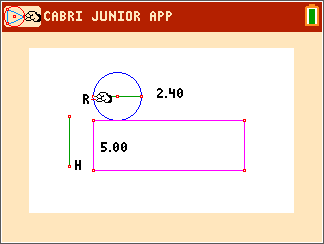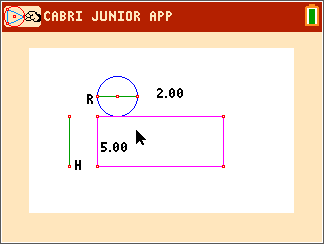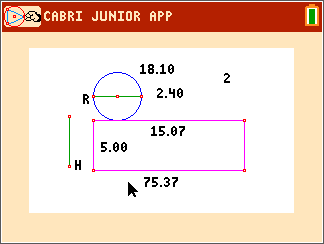••• ##### Device
• TI-84 Plus
• TI-84 Plus Silver Edition
•TI-84 Plus C Silver Edition
•TI-84 Plus CE
• ##### Software

TI Connect™ CE

# Geometry: Surface Area of a Cylinder

by Texas Instruments#### Overview

Students define right and oblique three dimensional figures and calculate the volume for prisms, pyramids, cylinders, and cones.

#### Key Steps

•In Problem 1, students use Cabri™ Jr. and open the file CYLINDER. The file shows a partial net for a right cylinder. If the rectangle of the net were rolled up, the circle would be the top face of the cylinder.

•They use D. & Length tool to find the length of the rectangle. Students use the Calculate tool to divide the length of the rectangle by the radius of the circle. The dimensions of the net can be changed, students drag the points R and H.

•Students use the Area tool from the Measure menu to find the areas of the rectangle and the circle.